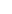This method typically takes advantage of the high volatility in a coin’s price during a 24-hour time period, it gives entry and exit values, but its disadvantage is that it does not take into account the trend that takes place. So let's say we plan to invest in the DigitalNote (XDN) coin. We open the Order Book and do the following calculations:

1. We Subtract the minimum 24-hour value from the maximum one: 0.00000355 -0.00000305 = 0.00000050
2. Then, we divide the result we found with 3: 0.00000050 / 3 = 0.00000017
3. The result we found is added to the minimum 24-hour price: 0.00000305 + 0.00000017 = 0.00000322
4. We place a purchase order between the minimum price and the value we previously calculated, i.e. from 0.00000305 to 0.00000322. Suppose our purchase order is placed at 0.00000312 and is filled.
5. We subtract the extra margin from the maximum allowed purchase price: 0.00000322 -0.00000312 = 0.00000010
6. We deduct the result found in (2) from the maximum 24 hour value: 0.00000355 -0.00000017 = 0.00000338
7. Finally, we subtract from the result found in (6) the result from (5): 0.00000338 -0.00000010 = 0.00000328.

The result we have found will be the appropriate selling price for this currency. It should be noted that depending on conditions and trends, we may change the selling price (since we still have profit), or even the ratios we use. For example, if we want to apply a more conservative tactic, we can divide the profit per unit by 2: (0.00000328 -0.00000312) / 2 = 0.00000016 / 2 = 0.00000008 and place the sell order at 0.00000328 -0.00000008 = 0.00000320.to signal pagePrice splitting method ❺❺❺ - average rating 5 from 5 (based on 191 user reviews)